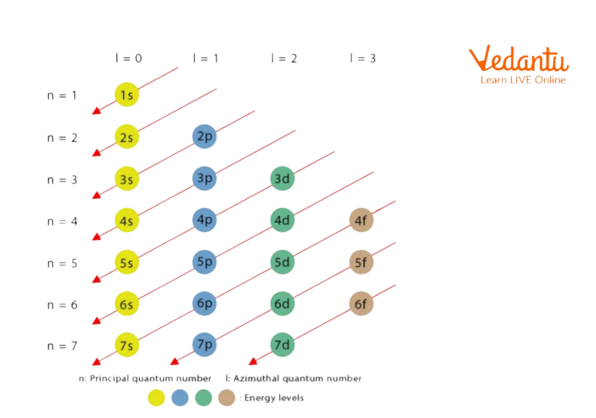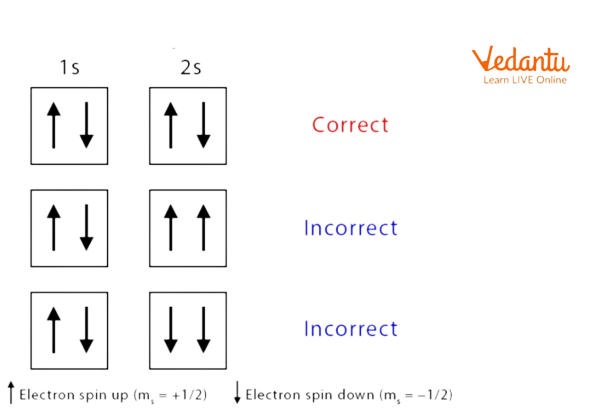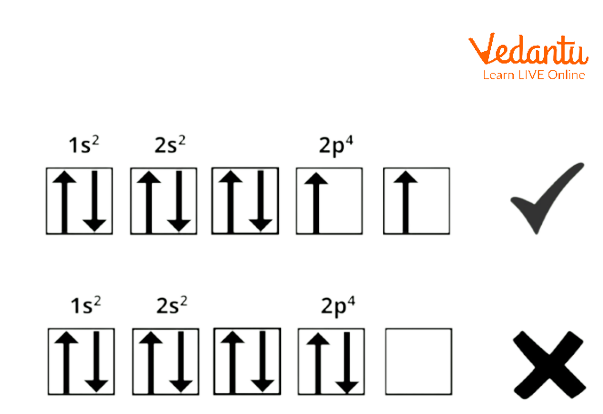Courses
Courses for Kids
Free study material
Free LIVE classes
More

# Electron Filling Rules## Filling of Electrons in an Orbital: Introduction

Last updated date: 15th Mar 2023
Total views: 63.6k
Views today: 1.43k

The filling of electrons in an orbital is the way to represent the arrangement of all the electrons in a specific atom. The electronic configuration of a molecule describes the positioning of electrons in a molecular orbital. The steadiness of an atom or a molecule can be determined with the means of electronic configuration. Each shell features a fixed number of electrons. It is determined by a simple formula, where the utmost number of electrons for the nth shell is 2n2. When all the orbitals of an atom are filled, it becomes an unreactive and stable atom.

## Rules For Filling Electrons In An Orbital

Rules to determine the positions of the electrons (electronic configuration) are :

1. Aufbau Principle

2. Pauli Exclusion Principle

3. Hund’s Rule

The filling of electrons into the orbitals of different atoms takes place according to the Aufbau principle which is based on Pauli’s exclusion principle, Hund’s rule of maximum multiplicity, and the relative energies of the orbitals.

## Aufbau Principle

Aufbau is a German word that means 'building up, and is not the name of a scientist unlike many of the other principles of chemistry. This principle is basically concerned with the filling of the electrons in an orbital during the writing of an electronic configuration.

'Building up’, as the name suggests, is regarding the filling of the orbitals with electrons in order to build the electronic configuration in a particular way so that an orbital with lower energy is filled earlier and the orbital with higher energy is filled later.

In other words, “In a ground state of the atoms, the orbitals are filled in order of their increasing energies." i.e. an electron will initially occupy an orbital of lower energy level and when the lower energy level orbitals are occupied, then only they shall start occupying the higher energy level orbitals.

## Features Of The Aufbau Principle

1. According to the Aufbau principle, electrons enter within the orbitals with the lowest energy 1st. This implies that electrons enter higher-energy orbitals solely once lower-energy orbitals are fully crammed or occupied.

2. The (n+l) rule will be used to confirm the sequence during which the energy of orbitals is occupied, where the total of the primary and azimuthal quantum numbers determines the energy state of the orbital.

3. Lower (n+l) values indicate the orbital with a lower energy state. If 2 orbitals have equivalent (n+l) values, the orbital with the lower n value is alleged to own lower energy.

4. Each and each orbital is full of electrons within the order: 1s, 2s, 2p, 3s, 3p, 4s, 3d, 4p, 5s, 4d, 5p, 6s, 4f, 5d, 6p, 7s, 5f, 6d, 7p, and so on.

The order in which the energies of the electronic orbitals increase and their respective order of filling as per the Aufbau rule is as follows:Order of Filling of an Electron by Aufbau Principle

This diagram shows the order of filling of electrons in its orbital.

For example Nitrogen (N)

Nitrogen has an atomic number = of 7 (the total number of electrons is 7)

According to the Aufbau principle, the filling of these seven electrons will follow the order as shown :

The first two electrons will enter in 1s orbital, the next 2 electrons will enter in 2s orbital (after the filling of the 1s orbital) and the remaining three electrons will enter the 2p orbital.

From this information, one can determine the valence shell, which will be the second shell(n=2). Further, the number of valence electrons will be 5.

The electronic configuration of the nitrogen atom can be written as 1s22s22p3.

The filling of the orbitals goes on according to the Aufbau rule/Aufbau principle. However, the location, the order of the filling of electrons on the basis of their spin while filling in the degenerate orbitals, and the spin of the 2 electrons filled in the same orbital itself are further considered by Hund’s rule and Pauli’s exclusion principle.

## Pauli Exclusion Principle

The principle was projected by nuclear physicists in 1925. According to Pauli's law, any 2 electrons in an atom will have an identical set of 4 quantum numbers(means they'll enter in the same orbital) but only 2 electrons could also be present within the same orbital and these electrons should have opposite spin.

## The Rules Of The Pauli’s Law

• 2 electrons will occupy identical orbits.

• 2 electrons that are present within the same orbital should have opposite spins or are parallel.

It is known as the exclusion principle. According to this principle if one electron in an atom has the same specific values for the four quantum numbers, then all the opposite electrons in this atom are excluded from having an identical set of values.Pauli Exclusion Principle

The above image shows that according to this principle the electrons must have a different spin and they must occupy the same orbitals.

## Hund’s Rule

Hund's rule states that:

1. Every orbital in a very sublevel is individually occupied before any orbital is doubly occupied.

2. All of the electrons in individually occupied orbitals have identical spin (to maximize total spin).

Hund's rule states that pairing of electrons within the orbitals of the same subshell doesn't occur till every orbital of that subshell has one electron per. as an example if there are 3 p, 5 d, and 7 f orbitals, therefore,  the pairing of electrons Starts from p, d, and f orbitals with fourth,6th, and eight electrons.Hund's Rule

Hund's rule is vital as a result of it specifies the order of filling the electron in a very set of the orbital. The orbital filling could be a secured diagram to point to electron location in a very completely different orbital.

## Summary

The filling of electrons into an orbital is the way to represent the arrangement of all the electrons in a specific atom. The different ways of filling electrons in the associated orbital of an atom are the Aufbau principle, Pauli law, and Hund’s rule. Hund's rule is significant as a result of it specifies the order of filling the electron in a {very} very set of the orbital. whereas the Aufbau principle helps in deciding the electronic configurations of components. The filling of orbitals may be useful in informing the position of electrons in several orbitals.

## FAQs on Electron Filling Rules

1. What is electronic configuration?

In Quantum Chemistry, an electronic configuration is the distribution of electrons in an associate atom or molecule (or different chemical entity). For instance, the negatron configuration of a noble gas atom is 1s22s22p6. This suggests that the 1s, 2s, and two subshells square measure occupied by 2, 2, and vi electrons, severally. The negatron configuration of the part represents however the electrons square measure distributed in their atomic orbitals. The negatron configuration of the associate atom follows the quality notation during which the associate atomic subshell containing all electrons is organized in sequence. For instance, the negatron configuration of metallic elements is 1s22s22p63s1.

2. What is the exception to the Aufbau principle?

The electron configuration of metal is [Ar]3d54s1 and not [Ar]3d44s2 (as instructed by the Aufbau principle). This exception is thanks to the many factors like the raised stability provided by half-filled subshells and therefore the comparatively low energy gap between the 3d and therefore the 4s subshells. Half-filled subshells faces lower electron-electron repulsions within the orbitals, thereby less increase in the energy gap. Similarly, fully filled subshells comparatively increase the energy gap of the atom. Therefore, the electronic configurations of some atoms donot follow the Aufbau principle (depending on the energy gap between the orbitals).

3. What are the limitations of the Aufbau principle?

The Aufbau principle can't be accustomed to predicting the electron configuration of atoms on ionization. In different words, it doesn't tell us that electrons square measure to be removed once associate particle types|is made|is created} form associate atom. though (n-1) d subshells and ns subshells lie quite close, nonetheless the previous is somewhat higher in energy. consequently the anticipated Aufbau outer configurations of metallic element (Z = 34) is .....3d45s2 however the by experimentation supported configuration is  ....3d54s1. just in the case of metal palladium (Z=46), the anticipated Aufbau configuration is 5s2 4d8 however the experimentation discovered configuration is 5s04d10.